# Legacy DocumentImportant: The information in this document is obsolete and should not be used for new development.

Inside Macintosh: QuickDraw GX Environment and Utilities /
Chapter 8 - QuickDraw GX Mathematics / Using QuickDraw GX Mathematics

## Converting Between Cartesian and Polar Coordinates

You can use QuickDraw GX functions to convert between Cartesian and polar coordinates. The `PolarToPoint` function converts a point in polar coordinates to Cartesian coordinates, (r, a) to (x, y). The `PointToPolar` function converts a point in Cartesian coordinates to polar coordinates, (x, y) to (r, a). The `gxPolar` point (r, a) corresponds to the `gxPoint` point (r cos(a), r sin(a)). Since r2 = x2 + y2 and tan(a) = y / x, the `gxPoint` structure (100, 100) corresponds to the `gxPolar` structure (141.42136, 45). Figure 8-19 shows the Cartesian coordinate of point (100, 100) and the polar coordinate of identical point (141.42136, 45).

Figure 8-19 Converting between Cartesian and polar coordinates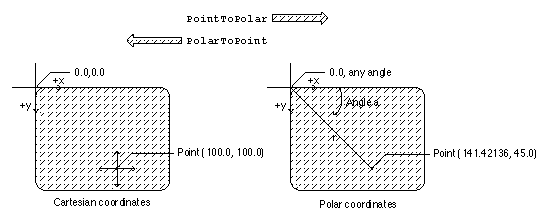The Cartesian and polar coordinate systems are described in the section "Cartesian and Polar Coordinate Conversion" beginning on page 8-10. The `PolarToPoint` function is described on page 8-56. The `PointToPolar` function is described on page 8-57.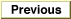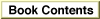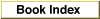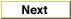© Apple Computer, Inc.
7 JUL 1996